# Boolean logic

Also found in: Dictionary, Thesaurus, Medical, Financial, Wikipedia.

(mathematics)

## Boolean logic

The "mathematics of logic," developed by English mathematician George Boole in the mid-19th century. Just as add, subtract, multiply and divide are the primary operations of arithmetic, AND, OR and NOT are the primary logical operators of Boolean logic and building blocks of every digital circuit. NAND (not AND), NOR (not OR) and XOR (exclusive OR) are variations of AND, OR and NOT (see NAND, NOR and XOR). See Boolean search, binary, logic gate and Bebop to the Boolean Boogie.

Decimal vs. Binary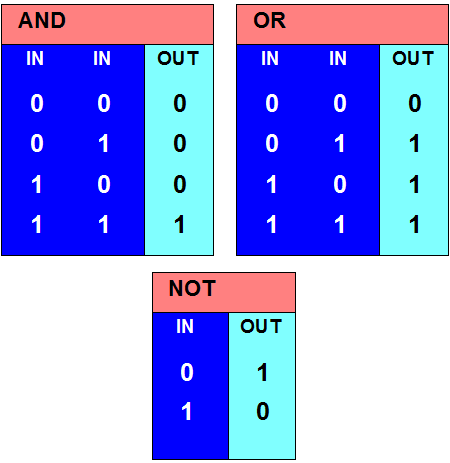Decimal arithmetic holds 10 values (0 to 9) in each digit position. In binary, there are only two (0 and 1). Note below the four possible result and carry bits when adding two binary digits (bits) together.

AND, OR and NOT Gates
Transistors wired in series and parallel patterns make up these "gates," which accept inputs of 0 (no pulse) or 1 (a pulse) and generate outputs of 0 or 1. While AND requires that both inputs are 1 to generate an output of 1, OR only needs one input to be 1. NOT reverses the input. For a diagram of these actions, see Boolean gates.

Decimal vs. Binary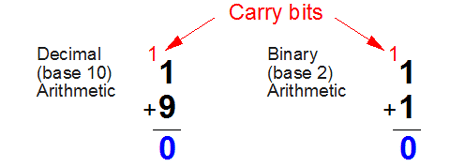Decimal arithmetic holds 10 values (0 to 9) in each digit position. In binary, there are only two (0 and 1). Note below the four possible result and carry bits when adding two binary digits (bits) together.

Add a One and Zero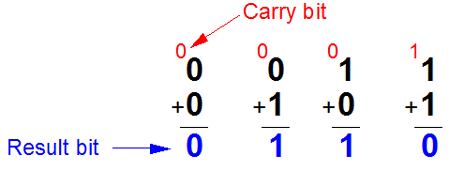Trace the red 1 (pulse, current) and white 0 (no pulse, no current) through the gates and notice their outputs. This half-adder circuit is in every CPU.

Add a One and Zero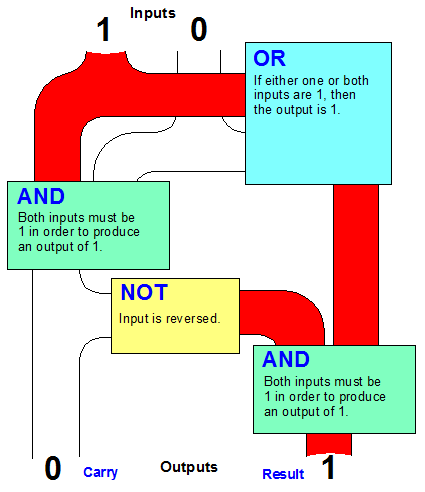Trace the red 1 (pulse, current) and white 0 (no pulse, no current) through the gates and notice their outputs. This half-adder circuit is in every CPU.

Trace the Flow YourselfChoose any two binary digits as input and trace them through this half-adder circuit (0 is no current; 1 is current). Watch how the output is generated.

Trace the Flow Yourself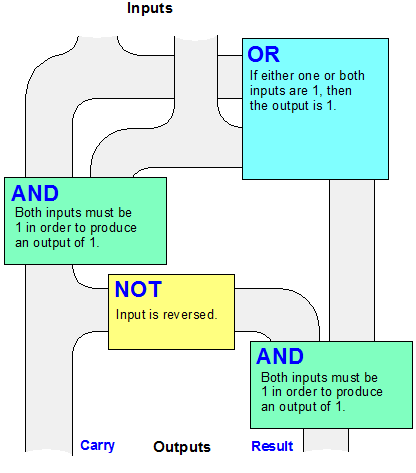Choose any two binary digits as input and trace them through this half-adder circuit (0 is no current; 1 is current). Watch how the output is generated.

Patterns of Boolean Logic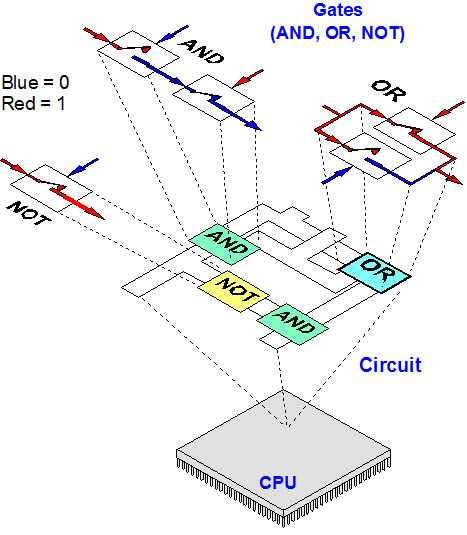Transistors (depicted here as mechanical switches) make up gates. Gates make up circuits, and circuits make up every digital device. There are millions and billions of transistors in modern chips, and they fit in an area smaller than a postage stamp. For more details, see Boolean gates. See transistor and chip.
Copyright © 1981-2019 by The Computer Language Company Inc. All Rights reserved. THIS DEFINITION IS FOR PERSONAL USE ONLY. All other reproduction is strictly prohibited without permission from the publisher.
References in periodicals archive ?
The Option nodes basically represent the OR boolean logic operations.
While a bias toward reductionist materialism and Boolean logic in the field of cognitive science has contributed a great deal in terms of understanding cognitive mechanisms, many problems remain unsolved.
Assertion proposition logic (AL) for PSL is defined as standard Boolean logic. A Boolean expression of AL is an expression that is evaluated in a single cycle and has the value true or false.
The book presupposes a good knowledge of advanced calculus and Boolean logic. The implications of constraint logic have much more to do with the development of artificial intelligence than cleaning up in Las Vegas, but for those who are interested in Martian rovers capable of making decisions; this is an exciting computational tool.
Use Boolean Logic. George Boole was a 19th-century English mathematician who helped establish the field of symbolic logic.
Fuzzy logic is derived from fuzzy set theory (Zadeh, 1965) dealing with reasoning that is approximate rather than precisely deduced from classical predicate logic (Boolean Logic).
The crisp set and Boolean logic approach as presented by Ragin (1987) and others (Alasuutari, 1995) is based on the qualitative tradition of focusing on cases and conditions within the cases and then using Boolean algebra to look at all the configuration of conditions to test relationships with specific outcomes.
The problem inherent in artificial intelligence is that it uses boolean logic, which is sequential and hierarchical.
The content, delivered through narration, text, and interactive exercises, includes lessons in binary numbers, Boolean logic, weirs and water flow, force, balance, and trajectory.
Lancaster is a master of clear explanation of concepts that are often unfamiliar or confusing to his readers, including Boolean logic, file structures, evaluation criteria, and vocabulary control.
We need a set of Boolean logic gates that can perform AND, OR, NOT and FANIN and FAN OUT  Operations.

Site: Follow: Share:
Open / Close